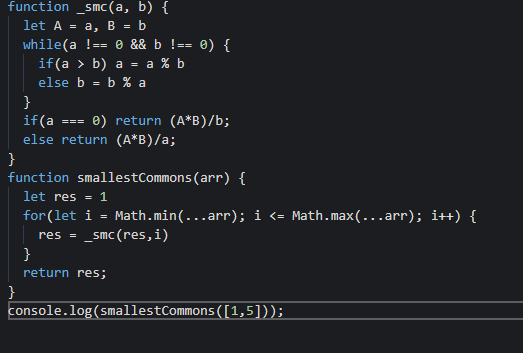# Algorithm Complexity: Smallest Common Multiple

Hi everyone, I 've just completed the above task in JS algorithm by creating a func to check SMC between 2 numbers and then run through the given array
Its quite simple but i still want to ask if there are any other solutions which have a shorter run-time since Im not good at Algorithm thinkingAnyway tks a lotSO I’m not REALLY going to answer your question. Well, ish. First, you’re getting the wrong answer. `smallestCommons([1,5])` should return 60, not 12. Your for loop is excluding the last member in the sequence.

But here’s a neat little trick you can use, thanks to the joys debugging: Using your same code, with a slight fix (the one I mentioned above), I added a line above and below the function caller:

``````function _smc(a, b){
let params = {a, b};
while( a !== 0 && b !== 0){
if (a>b) a = a  % b;
else b = b % a;
}
if (a === 0) return (params.a*params.b)/b
else return (params.a*params.b)/a
}

function smallestCommons(arr){
let min = Math.min(...arr),
max = Math.max(...arr);
res = min;
for (let i=(Math.min(...arr)); i <= Math.max(...arr); i++){
res= _smc(res, i)
}
return res;
}

console.time("SmallestCommon"); // <--I added this line...
console.log(smallestCommons([3, 20]));
console.timeEnd("SmallestCommon");  // <-- ...and this one.

``````

Now, in the console, you can see exactly how long your function actually takes. Change things, try different approaches, but leave those lines in. You can perform a pretty basic test for efficiency with that.